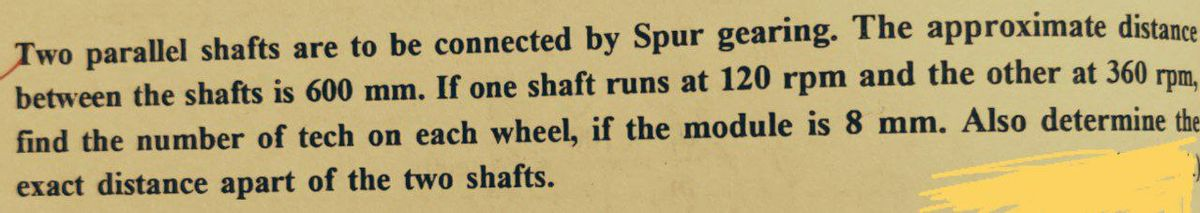# Two parallel shafts are to be connected by Spur gearing. The approximate distance between the shafts is 600 mm. If one shaft runs at 120 rpm and the other at 360 rpm. find the number of tech on each wheel, if the module is 8 mm. Also determine the exact distance apart of the two shafts.

Question-AnswerCategory: Kinematics of MachineTwo parallel shafts are to be connected by Spur gearing. The approximate distance between the shafts is 600 mm. If one shaft runs at 120 rpm and the other at 360 rpm. find the number of tech on each wheel, if the module is 8 mm. Also determine the exact distance apart of the two shafts.Two parallel shafts are to be connected by Spur gearing. The approximate distance between the shafts is 600 mm. If one shaft runs at 120 rpm and the other at 360 rpm. find the number of tech on each wheel, if the module is 8 mm. Also determine the exact distance apart of the two shafts.